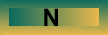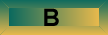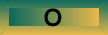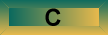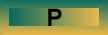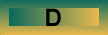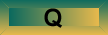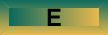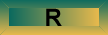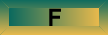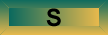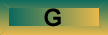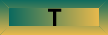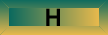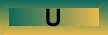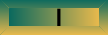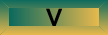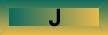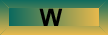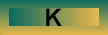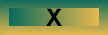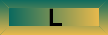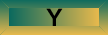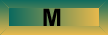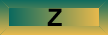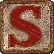State Space A state space is a theoretical representation of a system, where the system can be viewed as a point in some multidimensional space. The coordinates of the point are given by the current values of all of the system's parameters (e.g., if the system is a network, then the current values of all connection weights could locate the system in the space). In addition, another variable is usually plotted in the state space. For instance, the height of the space at the point's (system's) location is also usually plotted. To continue the network example, at the network's point in space, the network might be associated with a particular amount of overall error that could be represented as the height of the state space's surface at the network's position. State spaces are of interest because they are usually used to understand dynamic systems (Port & Van Gelder, 1995). A dynamic system is a system that changes over time. These changes can be represented in a state space as a trajectory -- a path, a set of positions -- on the represented surface. For instance, a Hopfield network is a dynamic system whose activation units change over time until the system stabilizes. When it stabilizes, recall has occurred. Recall can be represented in a state space as the path from the the location of the network in the space when a cue is presented to the location of the network in the space when it stabilizes. Stability would result when the network comes to rest in a local minimum. Thus learning and recall in a Hopfield network can be thought of as creating, and later finding, "valleys" in a state space that represents network energy or error. References: Hopfield, J. J. (1982). Neural networks and physical systems with emergent collective computational abilities. Proceedings of the National Academy of Sciences, 79, 2554-2558. Hopfield, J. J. (1984). Neurons with graded response have collective computational properties like those of two state neurons. Proceedings of the National Academy of Sciences USA, 81, 3008-3092. Port, R. F., & Van Gelder, T. (1995). Mind as motion: Explorations in the dynamics of cognition. Cambridge, Mass.: MIT Press. (Added January 2010)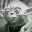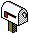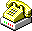(780)-492-5175
 WWW www.bcp.psych.ualberta.ca